# Mean spherical approximation

Jump to: navigation, search

The mean spherical approximation (MSA) closure relation of Lebowitz and Percus is given by :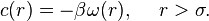$c(r) = -\beta \omega(r), ~ ~ ~ ~ r>\sigma.$

In the Blum and Høye mean spherical approximation for mixtures the closure is given by  :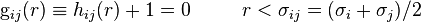${\rm g}_{ij}(r) \equiv h_{ij}(r) +1=0 ~ ~ ~ ~ ~ ~ ~ ~ r < \sigma_{ij} = (\sigma_i + \sigma_j)/2$

and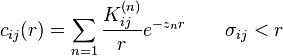$c_{ij}(r)= \sum_{n=1} \frac{K_{ij}^{(n)}}{r}e^{-z_nr} ~ ~ ~ ~ ~ ~ \sigma_{ij} < r$

where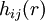$h_{ij}(r)$ and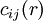$c_{ij}(r)$ are the total and the direct correlation functions for two spherical molecules of$i$ and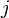$j$ species,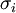$\sigma_i$ is the diameter of$i$ species of molecule. Duh and Haymet (Eq. 9 in ) write the MSA approximation as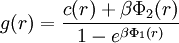$g(r) = \frac{c(r) + \beta \Phi_2(r)}{1-e^{\beta \Phi_1(r)}}$

where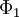$\Phi_1$ and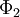$\Phi_2$ comes from the Weeks-Chandler-Andersen division of the Lennard-Jones potential. By introducing the definition (Eq. 10 in )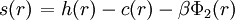$\left.s(r)\right. = h(r) -c(r) -\beta \Phi_2 (r)$

one can arrive at (Eq. 11 in )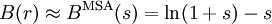$B(r) \approx B^{\rm MSA}(s) = \ln (1+s)-s$

The Percus Yevick approximation may be recovered from the above equation by setting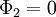$\Phi_2=0$.